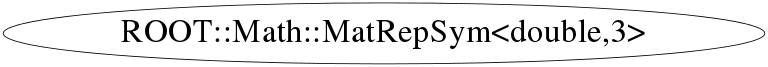# class ROOT::Math::MatRepSym<double,3>


MatRepStd
Standard Matrix representation for a general D1 x D2 matrix.
This class is itself a template on the contained type T, the number of rows and the number of columns.
Its data member is an array T[nrows*ncols] containing the matrix data.
The data are stored in the row-major C convention.
For example, for a matrix, M, of size 3x3, the data \f$\left[a_0,a_1,a_2,.......,a_7,a_8 \right] \f$d are stored in the following order:
\f[
M = \left( \begin{array}{ccc}
a_0 & a_1 & a_2  \\
a_3 & a_4  & a_5  \\
a_6 & a_7  & a_8   \end{array} \right)
\f]

@ingroup MatRep



## Function Members (Methods)

public:
 ~MatRepSym() double apply(unsigned int i) const double* Array() const double* Array() const ROOT::Math::MatRepSym MatRepSym() ROOT::Math::MatRepSym MatRepSym(const ROOT::Math::MatRepSym&) static int off(int i) static int off0(int i) static int off1(int i) static int off2(int i, int j) static unsigned int offset(unsigned int i, unsigned int j) double& operator()(unsigned int i, unsigned int j) const double& operator()(unsigned int i, unsigned int j) const ROOT::Math::MatRepSym& operator+=(const ROOT::Math::MatRepSym& rhs) ROOT::Math::MatRepSym& operator-=(const ROOT::Math::MatRepSym& rhs) ROOT::Math::MatRepSym& operator=(const ROOT::Math::MatRepSym& rhs) ROOT::Math::MatRepSym& operator= >(const ROOT::Math::MatRepSym&) double& operator[](unsigned int i) const double& operator[](unsigned int i) const

## Data Members

public:
 static ROOT::Math::MatRepSym::(anonymous) kCols static ROOT::Math::MatRepSym::(anonymous) kRows static ROOT::Math::MatRepSym::(anonymous) kSize
private:
 double fArray

## Class Charts## Function documentation

const T& operator()(unsigned int i, unsigned int j) const
T& operator()(unsigned int i, unsigned int j)
T& operator[](unsigned int i)
{ return fArray[i]; }
const T& operator[](unsigned int i) const
{ return fArray[i]; }
T apply(unsigned int i) const
{ return fArray[i]; }
T* Array()
{ return fArray; }
const T* Array() const
{ return fArray; }
MatRepStd<T, D1, D2>& operator+=(const ROOT::Math::MatRepSym<double,3>& rhs)
MatRepStd<T, D1, D2>& operator-=(const ROOT::Math::MatRepSym<double,3>& rhs)
MatRepStd<T, D1, D2>& operator=(const ROOT::Math::MatRepSym<double,3>& rhs)
return fArray[ off(int i)
constexpr int off0(int i)
{ return i==0 ? 0 : off0(i-1)+i;}
constexpr int off2(int i, int j)
{ return j<i ? off0(i)+j : off0(j)+i; }
constexpr int off1(int i)
{ return off2(i/D, i%D);}
offset(unsigned int i, unsigned int j)
if (j > i) std::swap(i, j);